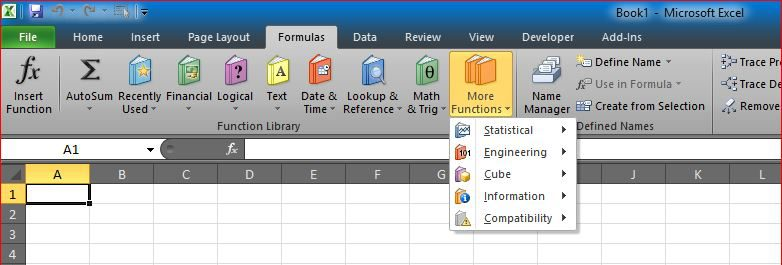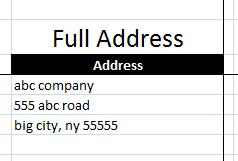Convert US addresses from cells (one column with 1 line for Name, 1 line for address, one line for City, State and Zip code) into table

Now, say you have multiple addresses in column B, structured as line per cell, like screenshot above

Which is usually what you got from any list of addresses online, and you want to convert it to table.

This is exactly what one of clients had and needed, so here is the file that does that.

Basically you need to have 5 columns with formula in column G as:

`=OFFSET(\$B\$1,(ROW()-5)*4+4,0)`

to extract first line (Starting from cell B5

Then in column H as:

`=OFFSET(\$B\$1,(ROW()-5)*4+5,0)`

Now, in column I you need to extract only city name, so you need this formula:

`=LEFT(OFFSET(\$B\$1,(ROW()-5)*4+6,0),SEARCH(",",OFFSET(\$B\$1,(ROW()-5)*4+6,0))-1)`

Then in J, use this formula:

`=MID(OFFSET(\$B\$1,(ROW()-5)*4+6,0),SEARCH(",",OFFSET(\$B\$1,(ROW()-5)*4+6,0))+2,2)`

To get state, then in K this:

`=MID(OFFSET(\$B\$1,(ROW()-5)*4+6,0),SEARCH(J5,OFFSET(\$B\$1,(ROW()-5)*4+6,0))+3,5)`

to get the Zipcode:

This way, you will convert all addresses into a table with address per row.